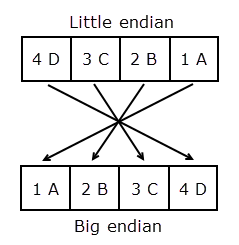# swapbytes

## 语法

``Y = swapbytes(X)``

## 说明

``Y = swapbytes(X)` 将数组 X 中每个元素的字节排序从 little endian 转换为 big endian（或相反）。输入数组的所有元素必须是完整的非复数数值元素。`

## 示例

```X = uint32(hex2dec('4D3C2B1A')); Y = dec2hex(swapbytes(X))```
```Y = '1A2B3C4D' ```

```X = 0x4D3C2B1A; Y = dec2hex(swapbytes(X))```
```Y = '1A2B3C4D' ```

```format short X = uint16([0 1 128 65535])```
```X = 1x4 uint16 row vector 0 1 128 65535 ```
`Y = swapbytes(X)`
```Y = 1x4 uint16 row vector 0 256 32768 65535 ```

```format hex X```
```X = 1x4 uint16 row vector 0000 0001 0080 ffff ```
`Y`
```Y = 1x4 uint16 row vector 0000 0100 8000 ffff ```

```format hex X = uint16(magic(3)*150); X(:,:,2) = X*40; X```
```X = 3x3x2 uint16 array X(:,:,1) = 04b0 0096 0384 01c2 02ee 041a 0258 0546 012c X(:,:,2) = bb80 1770 8ca0 4650 7530 a410 5dc0 d2f0 2ee0 ```
`Y = swapbytes(X)`
```Y = 3x3x2 uint16 array Y(:,:,1) = b004 9600 8403 c201 ee02 1a04 5802 4605 2c01 Y(:,:,2) = 80bb 7017 a08c 5046 3075 10a4 c05d f0d2 e02e ```

## 详细信息

### 将 Little Endian 转换为 Big Endian